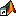MATLAB 启动文件夹

默认启动文件夹

Windows 平台上的默认文件夹

Windows 资源管理器工具中双击 matlab.exe 可执行文件

1. 右键点击 MATLAB 的快捷方式图标，然后从上下文菜单中选择属性。MATLAB 的“属性”对话框将在快捷方式窗格中打开。

2. 如果启动位置字段包含文本，请清除该文本。

3. 点击，然后再次尝试启动 MATLAB。

使用 userpath 作为初始工作文件夹

• 您可将所使用的 MATLAB 文件存储在一个文件夹中，例如 Documents/MATLAB

• 由于 MATLAB 自动将 userpath 文件夹添加到搜索路径顶层，因此始终可以运行文件。

• 首次运行新版 MATLAB 时，如果 userpath 文件夹不存在，软件会自动创建该文件夹。

• 当升级到新版 MATLAB 时，软件会自动继续使用相同的启动文件夹和现有文件。

• 默认 userpath 还可发挥 Windows 和 macOS 环境中用于存储个人文件的标准位置所具备的优势。当使用其他机器时，您仍然可以使用 Documents/MATLAB 文件夹中的文件。每个用户都有单独的 Documents/MATLAB 文件夹。其他用户（即使他们使用您的计算机）无法访问您的 Documents/MATLAB 文件夹中的文件。